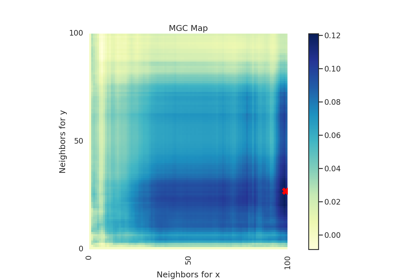# Hsic¶

class hyppo.independence.Hsic(compute_kernel='gaussian', bias=False, **kwargs)

Hilbert Schmidt Independence Criterion (Hsic) test statistic and p-value.

Hsic is a kernel based independence test and is a way to measure multivariate nonlinear associations given a specified kernel 1. The default choice is the Gaussian kernel, which uses the median distance as the bandwidth, which is a characteristic kernel that guarantees that Hsic is a consistent test 1 2.

Parameters
• compute_kernel (str, callable, or None, default: "gaussian") -- A function that computes the kernel similarity among the samples within each data matrix. Valid strings for compute_kernel are, as defined in sklearn.metrics.pairwise.pairwise_kernels,

["additive_chi2", "chi2", "linear", "poly", "polynomial", "rbf", "laplacian", "sigmoid", "cosine"]

Note "rbf" and "gaussian" are the same metric. Set to None or "precomputed" if x and y are already similarity matrices. To call a custom function, either create the similarity matrix before-hand or create a function of the form metric(x, **kwargs) where x is the data matrix for which pairwise kernel similarity matrices are calculated and kwargs are extra arguements to send to your custom function.

• bias (bool, default: False) -- Whether or not to use the biased or unbiased test statistics.

• **kwargs -- Arbitrary keyword arguments for compute_kernel.

Notes

The statistic can be derived as follows 1:

Hsic is closely related distance correlation (Dcorr), implemented in hyppo.independence.Dcorr, and exchanges distance matrices $$D^x$$ and $$D^y$$ for kernel similarity matrices $$K^x$$ and $$K^y$$. That is, let $$x$$ and $$y$$ be $$(n, p)$$ samples of random variables $$X$$ and $$Y$$. Let $$K^x$$ be the $$n \times n$$ kernel similarity matrix of $$x$$ and $$K^y$$ be the $$n \times n$$ be the kernel similarity matrix of $$y$$. The Hsic statistic is,

$\mathrm{Hsic}^b_n (x, y) = \frac{1}{n^2} \mathrm{tr} (D^x H D^y H)$

Hsic and Dcov are exactly equivalent in the sense that every valid kernel has a corresponding valid semimetric to ensure their equivalence, and vice versa 3 4. In other words, every Dcorr test is also an Hsic and vice versa. Nonetheless, implementations of Dcorr and Hsic use different metrics by default: Dcorr uses a Euclidean distance while Hsic uses a Gaussian median kernel. We consider the normalized version (see hyppo.independence) for the transformation.

The p-value returned is calculated using a permutation test using hyppo.tools.perm_test. The fast version of the test uses hyppo.tools.chi2_approx.

References

1(1,2,3)

Arthur Gretton, Kenji Fukumizu, Choon Teo, Le Song, Bernhard Schölkopf, and Alex Smola. A Kernel Statistical Test of Independence. Advances in Neural Information Processing Systems, 2007.

2

Arthur Gretton and László Györfi. Consistent Nonparametric Tests of Independence. Journal of Machine Learning Research, 11(46):1391–1423, 2010.

3

Cencheng Shen and Joshua T. Vogelstein. The exact equivalence of distance and kernel methods in hypothesis testing. AStA Advances in Statistical Analysis, September 2020. doi:10.1007/s10182-020-00378-1.

4

Dino Sejdinovic, Bharath Sriperumbudur, Arthur Gretton, and Kenji Fukumizu. Equivalence of distance-based and RKHS-based statistics in hypothesis testing. The Annals of Statistics, 41(5):2263–2291, October 2013. doi:10.1214/13-AOS1140.

Methods Summary

 Hsic.statistic(x, y) Helper function that calculates the Hsic test statistic. Hsic.test(x, y[, reps, workers, auto, ...]) Calculates the Hsic test statistic and p-value.

Hsic.statistic(x, y)

Helper function that calculates the Hsic test statistic.

Parameters

x,y (ndarray) -- Input data matrices. x and y must have the same number of samples. That is, the shapes must be (n, p) and (n, q) where n is the number of samples and p and q are the number of dimensions. Alternatively, x and y can be kernel similarity matrices, where the shapes must both be (n, n).

Returns

stat (float) -- The computed Hsic statistic.

Hsic.test(x, y, reps=1000, workers=1, auto=True, random_state=None)

Calculates the Hsic test statistic and p-value.

Parameters
• x,y (ndarray) -- Input data matrices. x and y must have the same number of samples. That is, the shapes must be (n, p) and (n, q) where n is the number of samples and p and q are the number of dimensions. Alternatively, x and y can be kernel similarity matrices, where the shapes must both be (n, n).

• reps (int, default: 1000) -- The number of replications used to estimate the null distribution when using the permutation test used to calculate the p-value.

• workers (int, default: 1) -- The number of cores to parallelize the p-value computation over. Supply -1 to use all cores available to the Process.

• auto (bool, default: True) -- Automatically uses fast approximation when n and size of array is greater than 20. If True, and sample size is greater than 20, then hyppo.tools.chi2_approx will be run. Parameters reps and workers are irrelevant in this case. Otherwise, hyppo.tools.perm_test will be run.

Returns

Examples

>>> import numpy as np
>>> from hyppo.independence import Hsic
>>> x = np.arange(100)
>>> y = x
>>> stat, pvalue = Hsic().test(x, y)
>>> '%.1f, %.2f' % (stat, pvalue)
'1.0, 0.00'


## Examples using hyppo.independence.Hsic¶